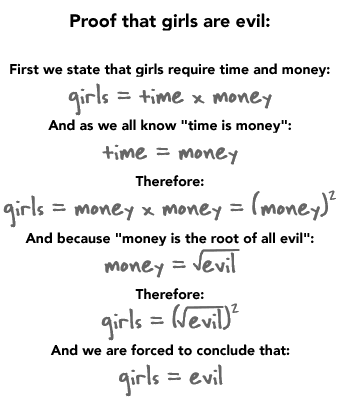# funny math problem

Status
Not open for further replies.

#### Pojo

Est. Contributor
I've seen that...Quite a bit...

#### Kip

##### Banned
I've never seen that before. It was kind of funny. Does anybody know what the answer is? I tried to solve it in my head, but I forget how to solve it. And it doesn't help that I'm horrible at algebra either.

#### starshine

Est. Contributor
I've never seen that before. It was kind of funny. Does anybody know what the answer is? I tried to solve it in my head, but I forget how to solve it. And it doesn't help that I'm horrible at algebra either.

[SIZE=+0]Pythagorean Theorem

x=3
[/SIZE]
²[SIZE=+0] + 4[/SIZE]²
[SIZE=+0] x=9+16
[/SIZE][SIZE=+0]x=(square root)25
x=5

[/SIZE]

#### Pojo

Est. Contributor
[SIZE=+0]Pythagorean Theorem

x=3
[/SIZE]
[SIZE=+0]squared + 4squared
x=9+16
[/SIZE][SIZE=+0]x=25
[/SIZE]

And then you square root it...So then it's 5...So x=5

#### starshine

Est. Contributor
And then you square root it...So then it's 5...So x=5

Darn I forgot that. Knew I was off.

fix'd

Est. Contributor

#### Pojo

Est. Contributor
That one is impossible to my brain. It has to be an optical illusion or something. I mean the triangles have to have the same area.

Chevre explained it to us in IRC...I could try to explain it to you on MSN if you want

#### recovery

Est. Contributor
[SIZE=+0]Pythagorean Theorem

x=3
[/SIZE]
²[SIZE=+0] + 4[/SIZE]²
[SIZE=+0] x=9+16
[/SIZE][SIZE=+0]x=(square root)25
x=5

[/SIZE]

Bah!?!? Don't you know your Pythagoras triplets?(whatever they are called)
3, 4, 5
5, 12, 13
7, 24, 25
8, 15, 17

As for the triangle one yes, i noticed that it wasn't a triangle either. I thought they cheated and modified one of the pieces. But upon closer inspection the gradient of one triangle did not match the other.

What are the roots of 8^(1/3) AKA the cube roots of 8.

#### Darkfinn

##### Banned
Here. Undeniable and definitive mathematical proof that girls are evil.Enjoy guys!

#### d4l

Est. Contributor
Status
Not open for further replies.